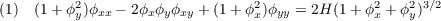#### Vol. 40, No. 2, 1972

 Download this articleFor screen For printingRecent Issues Vol. 325: 1  2 Vol. 324: 1  2 Vol. 323: 1  2 Vol. 322: 1  2 Vol. 321: 1  2 Vol. 320: 1  2 Vol. 319: 1  2 Vol. 318: 1  2Online Archive Volume: Issue:The Journal Subscriptions Editorial Board Officers Contacts Submission Guidelines Submission Form Policies for Authors ISSN: 1945-5844 (e-only) ISSN: 0030-8730 (print) Special Issues Author Index To Appear Other MSP Journals
A three point condition for surfaces of constant mean curvature

### Eamon Boyd Barrett

Vol. 40 (1972), No. 2, 269–277
##### Abstract

Let ϕ(x,y) be a solution to the equation:The quantity H in equation (1) represents the mean curvature of the surface z = ϕ(x,y). In case H = 0, (1) is the minimal surface equation. For minimal surfaces, the wellknown three point condition may be stated as follows:

Theorem 1. Let ϕ(x,y) be a solution to the Dirichlet problem for the minimal surface equation in some bounded region R. Let T be the continuous space curve defined by the values of ϕ(x,y) over ∂R, the boundary of R. Then, if P is a plane tangent to the surface z = ϕ(x,y) for (x,y) in R,P will have at least 4 points in common with T.

The objective of this paper is to establish a natural analogue of the three-point condition for surfaces of positive, constant mean curvature.

Primary: 53A10
##### Milestones